Students estimate quantities using rounding and develop fluency with the standard algorithm of addition and subtraction. Students focus on the precision of their calculations, and use them to solve real-world problems.

Math

Unit 1

## Unit Summary

In the first unit of 3rd grade, students will build on their understanding of the structure of the place value system from 2nd grade (MP.7) to estimate values by rounding them (3.NBT.1) and develop fluency with the standard algorithm of addition and subtraction (3.NBT.2). Throughout the unit, students attend to the precision of their calculations (MP.6) and use them to solve real-world problems (MP.4).

In 2nd grade, students developed an understanding of the structure of the base-ten system as based in repeated bundling in groups of 10. With this deepened understanding of the place value system, students "add and subtract within 1000, with composing and decomposing, and they understand and explain the reasoning of the processes they use" (NBT Progressions, p. 8). These processes and strategies include concrete models or drawings and strategies based on place value, properties of operations, and/or the relationship between addition and subtraction (2.NBT.7). As such, at the end of 2nd grade, students are able to add and subtract within 1,000 using a variety of strategies including algorithms, but are not yet fluent with them.

Because all students are not yet fluent with place value strategies, Unit 1 starts off by reinforcing some of the place value understanding that students learned in 2nd grade. Students use this sense of magnitude and the idea of benchmark numbers to first place numbers on number lines of various endpoints and intervals, and next use those number lines as a model to help them round two-digit numbers to the tens place as well as three-digit numbers to the hundreds and tens place (3.NBT.1). Next, students focus on developing their fluency with the addition and subtraction algorithms up to 1,000, making connections to the place value understandings and other models they learned in 2nd grade (3.NBT.2). Last, the unit culminates in a synthesis of all learning thus far in the unit, in which students solve one- and two-step word problems involving addition and subtraction and use rounding to assess the reasonableness of their answer (3.OA.8), connecting the NBT and OA domains. These skills are developed further and built upon in subsequent units in which students estimate and solve two-step word problems that also involve multiplication and division.

This builds toward an even deeper understanding of the place value system that students learn in 4th Grade Math. In 4th grade, students learn about multiplicative comparison, i.e., a value being x times as many as another value. Thus, students’ understanding of the place value system is more precisely refined as "a digit in one place represents ten times what it represents in the place to its right" (4.NBT.1, emphasis ours). Further, students learn to round any multi-digit number to any place. They also use the standard algorithm to solve addition and subtraction problems to the new place values they encounter at this grade level, namely, to one million. While the majority of the content learned in this unit is additional cluster content, they are deeply important skills necessary to be proficient with the major work of the grade with 3.OA.8, as well as a foundation for rounding and the standard algorithms used to any place value learned in 4th grade (4.NBT.1—4) and depended on for many grade levels after that.

Pacing: 16 instructional days (14 lessons, 1 flex day, 1 assessment day)

Fishtank Plus for Math

Unlock features to optimize your prep time, plan engaging lessons, and monitor student progress.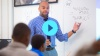## Assessment

The following assessments accompany Unit 1.

### Pre-Unit

Have students complete the Pre-Unit Assessment and Pre-Unit Student Self-Assessment before starting the unit. Use the Pre-Unit Assessment Analysis Guide to identify gaps in foundational understanding and map out a plan for learning acceleration throughout the unit.

### Mid-Unit

Have students complete the Mid-Unit Assessment after lesson 7.

### Post-Unit

Use the resources below to assess student understanding of the unit content and action plan for future units.

Expanded Assessment Package

Use student data to drive your planning with an expanded suite of unit assessments to help gauge students’ facility with foundational skills and concepts, as well as their progress with unit content.

## Unit Prep

### Intellectual Prep

Unit Launch

Prepare to teach this unit by immersing yourself in the standards, big ideas, and connections to prior and future content. Unit Launches include a series of short videos, targeted readings, and opportunities for action planning.#### Intellectual Prep for All Units

• Read and annotate "Unit Summary" and "Essential Understandings" portion of the unit plan.
• Do all the Target Tasks and annotate them with the "Unit Summary" and "Essential Understandings" in mind.
• Take the Post-Unit Assessment.

#### Unit-Specific Intellectual Prep

• Read the following table that includes models used in this unit.
Pictorial or concrete base ten blocks

Example: Represent 342 with base ten blocks.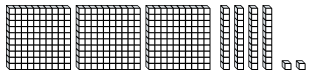Place value chart
 Ten thousands Thousands Hundreds Tens Ones 4 8 0 5 0
Number line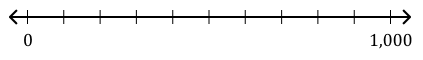Standard algorithm for addition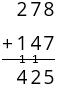Standard algorithm for subtraction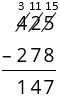Tape diagram

Example: A grocery store sells 172 red apples and 86 green apples. How many apples did the grocery store sell?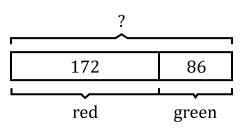### Essential Understandings

• When rounding a number, the goal is to approximate the number by the closest number with no units of smaller value (e.g., so 456 rounded to the nearest ten is 460 and to the nearest hundred is 500). When a number that is being rounded has a 5 in the place being considered and 0 in all smaller places, it is equidistant from the two benchmarks. Therefore, it is simply a convention that the number is rounded to the greater benchmark.
• Fluently adding and subtracting depends on a deep knowledge of the place value system and flexibility in solving. The numbers themselves should dictate which strategy is used, as sometimes that may be the standard algorithm, and other times it may be a mental strategy (such as converting 199 + 456 to 200 + 455).
• The standard algorithm for addition and subtraction is based on the idea of needing to add like-units together and the idea that one can regroup 1 of any unit to be 10 of the next smallest unit (and vice versa).
• Rounding values before computing with them can result in an estimate that is either too high or too low, depending on how the numbers were originally rounded, what computation is being performed, and where in the equation or expression it’s located.
• Rounding numbers can help one to determine whether an answer is reasonable, based on whether the estimate is close to the computed answer or not.
• Making sense of problems and persevering in solving them is an important practice when solving word problems. Key words do not always indicate the correct operation.

### Vocabulary

algorithm

approximate/approximation

approximately equal sign, $${\approx}$$

digit

estimate/estimation

place

reasonable

round

value

To see all the vocabulary for Unit 1, view our 3rd Grade Vocabulary Glossary.

### Materials

• Tic-Tac-Toe Boards (1 per pair of students)
• Optional: Markers or crayons (2 of different colors per pair of students) — Students could use a pen and a pencil instead.
• Optional: Paper base ten blocks (Total of 9 per student or small group) — Students might not need these depending on their reliance on concrete materials.
• Optional: Base ten blocks (Maximum of about 5 hundreds, 20 tens, 20 ones per student or small group) — Students might not need these depending on their reliance on concrete materials. Or, students can use Paper base ten blocks cut into units instead.
• Thousands Place Value Chart (Total of 6 per student) — Students might need more or less depending on their reliance on this tool.

## Unit Practice

Word Problems and Fluency Activities

Access daily word problem practice and our content-aligned fluency activities created to help students strengthen their application and fluency skills.## Lesson Map

Topic A: Foundations of Place Value

Topic B: Rounding to the Nearest Ten and Hundred

Topic C: Addition and Subtraction Within 1,000

## Common Core Standards

Key

Major Cluster

Supporting Cluster

### Core Standards

#### Number and Operations in Base Ten

• 3.NBT.A.1 — Use place value understanding to round whole numbers to the nearest 10 or 100.
• 3.NBT.A.2 — Fluently add and subtract within 1000 using strategies and algorithms based on place value, properties of operations, and/or the relationship between addition and subtraction.

#### Operations and Algebraic Thinking

• 3.OA.D.8 — Solve two-step word problems using the four operations. Represent these problems using equations with a letter standing for the unknown quantity. Assess the reasonableness of answers using mental computation and estimation strategies including rounding. This standard is limited to problems posed with whole numbers and having whole-number answers; students should know how to perform operations in the conventional order when there are no parentheses to specify a particular order (Order of Operations).

• 2.MD.B.6

• 2.NBT.A.1
• 2.NBT.A.2
• 2.NBT.A.3
• 2.NBT.B.5
• 2.NBT.B.7
• 2.NBT.B.8
• 2.NBT.B.9

• 2.OA.A.1

• 4.NBT.A.3
• 4.NBT.B.4

### Standards for Mathematical Practice

• CCSS.MATH.PRACTICE.MP1 — Make sense of problems and persevere in solving them.

• CCSS.MATH.PRACTICE.MP2 — Reason abstractly and quantitatively.

• CCSS.MATH.PRACTICE.MP3 — Construct viable arguments and critique the reasoning of others.

• CCSS.MATH.PRACTICE.MP4 — Model with mathematics.

• CCSS.MATH.PRACTICE.MP5 — Use appropriate tools strategically.

• CCSS.MATH.PRACTICE.MP6 — Attend to precision.

• CCSS.MATH.PRACTICE.MP7 — Look for and make use of structure.

• CCSS.MATH.PRACTICE.MP8 — Look for and express regularity in repeated reasoning.

Unit 2

Multiplication and Division, Part 1

## Request a Demo

See all of the features of Fishtank in action and begin the conversation about adoption.

Yes

No

### Any other information you would like to provide about your school?